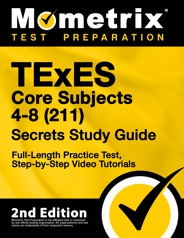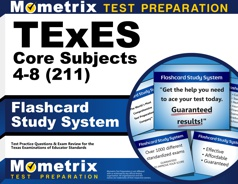# TExES Mathematics 4-8 (115) Practice Test

The TExES Mathematics 4-8 (115) exam is administered for those interested in becoming an entry-level educator within the Texas public school system, specifically for teaching 4th-8th-grade Mathematics.

Click “Start Test” below to take a free TExES Mathematics 4-8 practice test!

## TExES Mathematics 4-8 (115) Exam Outline

The TExES Mathematics 4-8 (115) exam contains 100 multiple-choice questions and has a time limit of 4 hours and 45 minutes.

The questions are split into six sections:

### I. Number Concepts (16%)

The questions in this section assess your knowledge of the following topics:

• The structure of number systems
• The relationship between quantity and symbolic representations
• Number operations
• Computational algorithms
• Number theory
• Using numbers to solve math and non-math problems

### II. Patterns and Algebra (21%)

The questions in this section assess your knowledge of the following topics:

• Using mathematical reasoning to identify and analyze patterns
• The relationships among variables, equations, relations, functions, expressions, and inequalities
• Solving problems using linear functions
• Solving problems using nonlinear functions and relations
• The conceptual foundations of calculus

### III. Geometry and Measurement (21%)

The questions in this section assess your knowledge of the following topics:

• Measurement as a process
• Geometric relationships and axiomatic structure of Euclidian geometry
• Properties of two- and three-dimensional figures
• Tranformational geometry
• Relating geometry to trigonometry

### IV. Probability and Statistics (16%)

The questions in this section assess your knowledge of the following topics:

• Using graphical and numerical techniques to explore data
• Theory of probability
• Relationship among probability theory, sampling, and statistical inference
• How statistical inference is used in making and evaluating predictions

### V. Mathematical Processes and Perspectives (10%)

The questions in this section assess your knowledge of the following topics:

• Mathematical reasoning and problem-solving
• Communicating mathematical ideas and concepts

### VI. Mathematical Learning, Instruction, and Assessment (16%)

The questions in this section assess your knowledge of the following topics:

• How children learn and develop mathematical skills and concepts
• How to plan, organize, and implement instruction
• Using formal and informal assessment techniques to monitor and guide instructionCheck Out Mometrix's TExES Mathematics 4-8 Study Guide

Get practice questions, video tutorials, and detailed study lessons## TExES Mathematics 4-8 (115) Registration

To register for the exam, you must create an online NES account via their website. Once your account has been created, you can use it to register for the exam and schedule an exam date.

When you register, you will need to pay the $116 examination fee. ## Test Day You should arrive at the testing center at least 30 minutes before your scheduled exam time. Once you arrive, you will be asked to present one form of valid, government-issued photo ID. After the check-in process, you will be asked to leave all personal items in a locker outside the testing area. If you wear eyeglasses, they may be inspected at this time. Once you enter the testing room, you will be asked to sign an NDA, and you will be given a brief tutorial on the computer-adaptive testing system. Once the tutorial is complete, the exam will begin. You are allowed to take a restroom break during the exam, but the timer will not be paused while you are away. ## How the Exam is Scored The TExES Mathematics 4-8 (115) exam is computer-adaptive. Here’s a look at how it works:The first question is judged to be of medium difficulty, and depending on your performance, the next question may be easier or harder. If you do well on the first question, the second question will be harder; conversely, if you do poorly on the first question, the second question will be easy. The questions on this exam are rated on a scale of 100-300. The higher a question is rated, the harder the question is (e.g., a question marked as 235 will be more difficult than a question marked 135). To pass the exam, your final question must be on or above the 240 mark. If your final question is below this mark, you will not pass the exam, even if you have answered some questions above the 240 mark at some point during the exam. This is because the computer has rated the difficulty of your final question based on how you answered the previous questions. Check Out Mometrix's TExES Mathematics 4-8 Flashcards Get complex subjects broken down into easily understandable concepts## FAQs ### How long is the TExES Mathematics 4-8 (115) exam? The time limit for the exam is 4 hours and 45 minutes. ### How many questions are on the TExES Mathematics 4-8 (115) exam? There are 100 multiple-choice questions on the exam. ### What is the passing score for the TExES Mathematics 4-8 (115) exam? To pass the exam, you must achieve a scaled score of 240. ### How much does the TExES Mathematics 4-8 (115) exam cost? The examination fee is$116.By Eric Richter

Eric is the Product Development Manager responsible for developing and updating the Mometrix Teacher Certification and K-12 products. Eric has a bachelor’s degree in Sociology and a master’s of education in Teaching ESOL.

by Mometrix Test Preparation | This Page Last Updated: September 22, 2023

Get Actionable Study Tips
Join our newsletter to get the study tips, test-taking strategies, and key insights that high-performing students use.
Get Actionable Study Tips
Join our newsletter to get the study tips, test-taking strategies, and key insights that high-performing students use!